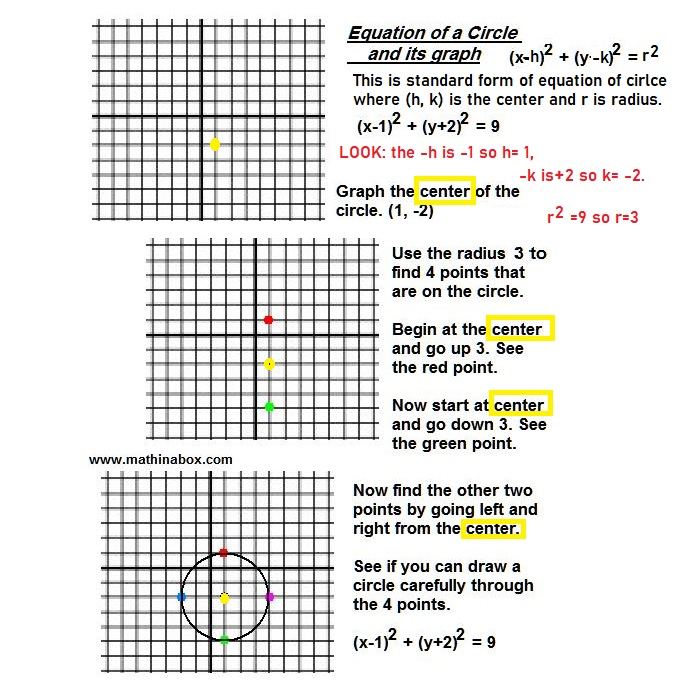# Graphing a Circle

Posted on: April 15, 2020 Posted by: Susan Johnsey Comments: 0(x +4)2 + (y – 12)2= 36 is the standard equation of a circle whose center is (-4, 12 ) and radius is 6.   NOTICE the opposite values of the center are used in the equation. Also, for the equation the radius, r, is squared.

Graph the center (-4, 12). Do you recall that is in the 2nd quadrant?

Then graph 4 points by starting at the center each time. Go up 6 for the first point. Again from the center go down 6. Repeat two more times: go left and then also right.

Draw the circle through the 4 points. Test one of your 4 points. What do I mean? If a point is on the circle then it will make the equation TRUE. If you started at (-4,12) and went up 6 then you should have the point (-4, 18). Now use x= – 4 and y=18 in the equation, will it make a TRUE math expression? You will have only numbers so it should be easy to decide as long as you recall the Order of Operations.

(x +4)2 + (y – 12)2 = 36 Let x= – 4 and y=18 and we get

(-4 +4)2 + (18 – 12)2 = 36. We will get 0 + 36 = 36. This is true so we know the point (-4, 18) is correct. I will let you test the other 3 points that you found.